Courses

# Study Notes for Cams Notes | EduRev

## Mechanical Engineering : Study Notes for Cams Notes | EduRev

The document Study Notes for Cams Notes | EduRev is a part of the Mechanical Engineering Course Theory of Machines (TOM).
All you need of Mechanical Engineering at this link: Mechanical Engineering

Cam

A cam is a mechanical member used to impart desired motion (displacement) to a follower by direct contact (either point or line contact). A cam may be rotating or reciprocating whereas the follower may be rotating, reciprocating or oscillating.

• Cam mechanisms belong to higher pair mechanism.
• A driver member known as cam.
• A driven member called the follower.
• A frame is one which supports the cam and guides the follower.
Definitions Related to Cam

The following terminologies are used to draw cam profile:

• Base circle: It is the smallest circle that can be drawn tangential to the cam profile (contour) from the centre of rotation of a radial cam. The base circle decides the overall size of a cam and is, therefore, a fundamental feature of the cam.
• Trace point: It is a reference point on the follower and is used to generate the pitch curve. In case of knife edge follower, the knife edge represents the trace point and in a roller follower, the centre of the roller represents the trace point.
• Pitch curve: It is the curve generated by the trace point assuming that the cam is fixed, and the trace point of the follower rotates around the cam i.e. the follower moves relative to the cam. For a knife edge follower, the pitch curve and the cam profile are same whereas for a roller follower, they are separated by the radius of the roller.
• Pressure angle: The pressure angle, representing the steepness of the cam profile, is the angle between the normal to the pitch curve at a point and the direction of the follower motion. During a complete rotation, the pressure angle varies from its maximum to its minimum value. The greater the pressure angle, the higher will be the thrust, and consequently chances of jamming of the translating follower in its guide will increase. In an offset follower, the pressure angle reduces during the follower rise but at the cost of increased pressure angle during the return stroke.
• Pitch point: It is a point on the pitch curve having the maximum pressure angle.
• Pitch circle: It is the circle passing through the pitch and concentric with the base circle.
• Prime circle: It is the smallest circle that can be drawn from the centre of the cam and tangent to the pitch curve. For a knife edge and a flat face follower, the prime circle and the base circle are identical. For a roller follower, the prime circle is larger than the base circle by the radius of the roller.
• Lift or stroke: It is the maximum travel of the follower from its lowest position to the topmost position.
• Common Normal: Line joining cam centre to the corresponding point of contact with cam denotes common normal to the roller surface and cam surface.
• Angle of Ascent: It is the angle through which the cam turns during the time the follower rises.
• Angle of Dwell: It is the angle through which the cam turns while the follower remains stationary at the highest or the lowest position.
• Angle of descent: It is the angle through which the cam turns during time the follower returns to the initial position.
Key Points
• For a roller follower, the trace point is at the roller centre.
• For a flat-face follower, it is at the point of contact between the follower and cam surface when the contact is along the base circle of the cam.
• During a complete rotation, the pressure angle varies from its maximum to its minimum value.
• The greater the pressure angle, the higher will be side thrust and consequently the changes of the translating follower jamming in its guide will increase.
• It is not desirable to increase the pressure angle.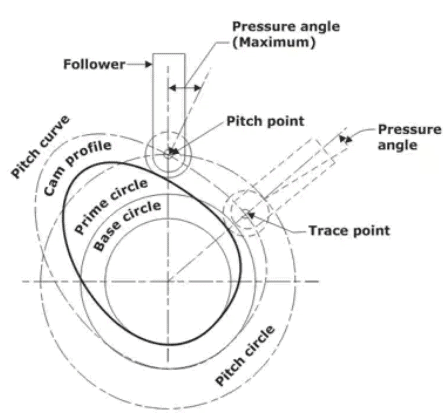Follower Displacement Diagram

The following terms are used with reference to the angular motion of the cam:

• Angle of Ascent (ϕa): It is the angle through which the cam turns during the time the follower rises.
• Angle of Dwell (f): Angle of dwell is the angle through which the cam turns while the follower remains stationery at the highest or the lowest position.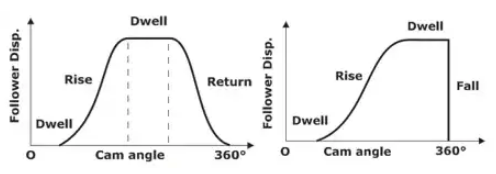• Angle of Descent (ϕd): Angle of descent is the angle through which the cam turns while follower returns to the initial position.
• Angle of Action: Angle of action is the total angle moved by the cam during the time between the beginning of rise and the end of return of the follower.
• In a specific interval of time, acceleration must be given to consider the velocity.
• The dynamic effect of acceleration limit the speed and effect of jerk (rate of change of acceleration) produce vibrations of the system.
• The angle made by follower to the surface of cam at the point of contact cannot be reduced from a certain value. So, it exerts minimum lateral pressure on the bearing.
• The size of the base circle controls the pressure angle.

Motion of the Follower

Though the follower can be made to have any type of desired motion, knowledge of the existing motion saves time and labour while designing the cams.

1. Uniform velocity or Constant Velocity
Constant velocity of the follower implies that the displacement of the follower is proportional to the cam displacement and the slope of the displacement curve is constant
Mathematically, Displacement of the follower given by
sαθ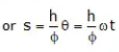θ is the cam angle of rotation in radians.
ϕ = outstroke angle of cam rotation,
s = stroke length of follower
For velocity differentiating with respective to time on both side of equation
(ds/dt) = V = (h/ϕ) (dθ/dt) = hω/ϕ (constant)
2. Uniform Acceleration and Retardation (Parabolic)
In such a follower programme, there is acceleration in the first half of the follower motion whereas it is deceleration during the later half. The displacement curve is found to be parabolic in this case. The magnitude of the acceleration and the deceleration is the same and constant in the two halves.
The equation for the linear motion with constant acceleration f (during the first half of the follower motion) is found as follows:
s = Vot + 1/2 ft2
Where vo is the initial velocity at the start of the motion (rise or fall) and is zero in this case,
⇒ s = 1/2 ft2
⇒ f = 2S/t2 = constant
As f is constant during the accelerating period, considering the follower at the midway.
s = h/2 and t = (ϕ/2)/ω
⇒ f = (2h/2)/(ϕ2/4ω2) = 4hω22
The velocity is linear during the period and is given by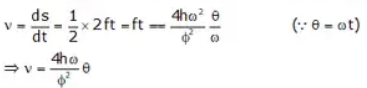The velocity is maximum when θ is maximum or the follower is at the midway, i.e. when θ = φ/2
⇒ vmax = (4hω/ϕ2) (ϕ/2) = 2hω/ϕ
3. Simple Harmonic motion
This is a popular follower motion and is easy to lay out.
Let
s = follower displacement (instantaneous)
h = maximum follower displacement
v = velocity of the follower
f = acceleration of the follower
θ = cam rotation angle (instantaneous)
ϕ = cam rotation angle for the maximum follower displacement
β = angle on the harmonic circle.

Displacement:
At any instant, displacement of the following is given by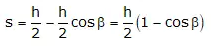Velocity: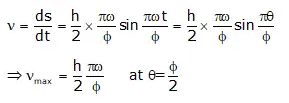Acceleration: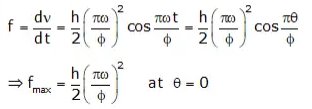Offer running on EduRev: Apply code STAYHOME200 to get INR 200 off on our premium plan EduRev Infinity!

## Theory of Machines (TOM)

94 videos|41 docs|28 tests

,

,

,

,

,

,

,

,

,

,

,

,

,

,

,

,

,

,

,

,

,

;